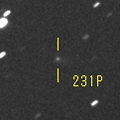# \$B%j%K%"(B-\$B%K!<%HWB@1(B

231P/LINEAR-NEAT (2019)###\$B###\$B50F;MWAG(B

```Epoch 2019 June 6.0 TT = JDT 2458640.5
T 2019 June 14.24642 TT                                 Rudenko
q   3.0224756            (2000.0)            P               Q
n   0.12241792     Peri.   42.74952     -0.98583510     -0.06120692
a   4.0170391      Node   133.02987     +0.01965560     -0.96677833
e   0.2475862      Incl.   12.33413     +0.16656176     -0.24818013
P   8.05
From 443 observations 1950 Apr. 20-2020 June 22, mean residual 0".8.
Nongravitational parameters A1 = +16.22, A2 = -0.7136.
```

###\$B8wEYJQ2=(B

```        m1 = 4.0 + 5 log\$B&\$(B + 25 log r
```##### \$B50F;MWAG\$O(BM.P.E.C. 2020-M114\$B\$K7G:\\$5\$l\$?\$b\$N\$G\$9!#(B \$B8wEY%0%i%U\$O(BComet for Windows\$B\$G:n@.\$7\$?\$b\$N\$G\$9!#(B